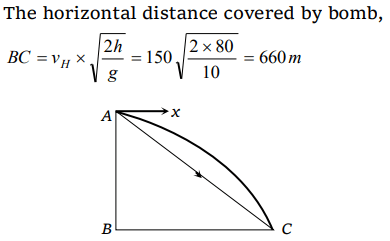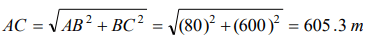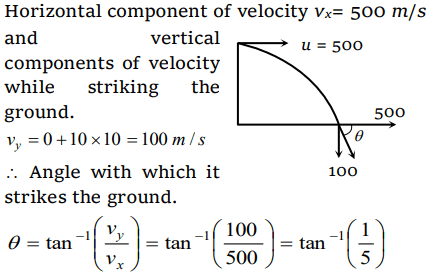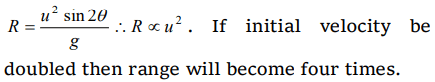## Motion in a Plane Questions and Answers Part-18

1. An aeroplane moving horizontally with a speed of 720 km/h drops a food pocket, while flying at a height of 396.9 m. the time taken by a food pocket to reach the ground and its horizontal range is $\left(g = 9.8m s^{-2}\right)$
a) 3 sec and 2000 m
b) 5 sec and 500 m
c) 8 sec and 1500 m
d) 9 sec and 1800 m

Explanation:2. A particle (A) is dropped from a height and another particle (B) is thrown in horizontal direction with speed of 5 m/sec from the same height. The correct statement is
a) Both particles will reach at ground simultaneously
b) Both particles will reach at ground with same speed
c) Particle (A) will reach at ground first with respect to particle (B)
d) Particle (B) will reach at ground first with respect to particle (A)

Explanation: For both cases t = $\sqrt{\frac{2h}{g}} = constant$
Because vertical downward component of velocity will be zero for both the particles

3. A particle moves in a plane with constant acceleration in a direction different from the initial velocity. The path of the particle will be
a) A straight line
b) An arc of a circle
c) A parabola
d) An ellipse

Explanation: A parabola

4. At the height 80 m, an aeroplane is moving with 150 m/s. A bomb is dropped from it so as to hit a target. At what distance from the target should the bomb be dropped $\left(g = 10m\diagup s^{2}\right)$
a) 605.3 m
b) 600 m
c) 80 m
d) 230 m

Explanation:The distance of target from dropping point of bomb,5. A bomber plane moves horizontally with a speed of 500 m/s and a bomb released from it, strikes the ground in 10 sec. Angle at which it strikes the ground will be $\left(g=10m\diagup s^{2}\right)$
a) $\tan^{-1}\left(\frac{1}{5}\right)$
b) $\tan\left(\frac{1}{5}\right)$
c) $\tan^{-1}\left(1\right)$
d) $\tan^{-1}\left(5\right)$

Explanation:6. A projectile fired with initial velocity u at some angle $\theta$ has a range R . If the initial velocity be doubled at the same angle of projection, then the range will be
a) 2R
b) $R\diagup2$
c) R
d) 4R

Explanation:7. If the initial velocity of a projectile be doubled, keeping the angle of projection same, the maximum height reached by it will
a) Remain the same
b) Be doubled
d) Be halved

Explanation:8. In the motion of a projectile freely under gravity, its
a) Total energy is conserved
b) Momentum is conserved
c) Energy and momentum both are conserved
d) None is conserved

Explanation: An external force by gravity is present throughout the motion so momentum will not be conserved

9. The range of a projectile for a given initial velocity is maximum when the angle of projection is $45^{\circ}$ . The range will be minimum, if the angle of projection is
a) $90^{\circ}$
b) $180^{\circ}$
c) $60^{\circ}$
d) $75^{\circ}$a) $45^{\circ}$
b) $\theta=\tan^{-1}\left(0.25\right)$
c) $\theta=\tan^{-1}4$   or $\left(\theta=76^{\circ}\right)$
d) $60^{\circ}$